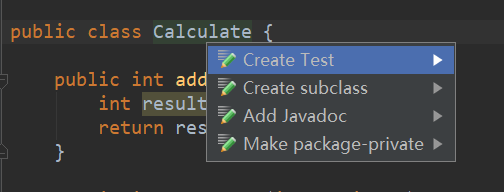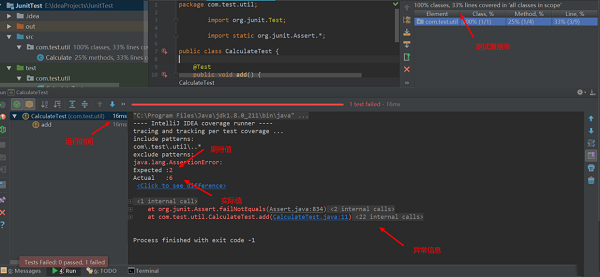junit单元测试步骤都有哪些？使用idea进行junit单元测试

TheDisguiser 2020-06-22 23:24:22 java常见问答 6389

Idea 默认是使用的 arquillian junit4 作为测试框架，这里我们我们把它改为 Junit4。

Settings -> Plugins -> 搜索栏中搜索 Junit 并勾选 -> 安装完成

Calculate.java

package com.test.util;
public class Calculate
{
public int add(int a, int b)
{
int result = a + b;
return result;
}
public int subtract(int a, int b)
{
int result = a - b;
return result;
}
public int multiply(int a, int b)
{
int result = a * b;
return result;
}
public int divide(int a, int b)
{
int result = a / b;
return result;
}
}

1)、新建一个名为 test 的目录

2)、右键该目录选择 Make Directory as -> Test Source Root

Idea 自动生成package com.test.util;
import org.junit.Test;
import static org.junit.Assert.*;
public class CalculateTest
{
@Test
{
assertEquals(2, new Calculate()
}
}junit单元测试idea，idea使用junit4单元测试实例

java junit单元测试实例，junit单元测试例子